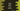# 3 ways in Java to print the Arithmetic Progression(AP) series## Java program to print the Arithmetic Progression or AP series:

In this post, we will learn how to print the Arithmetic Progression series in Java. An arithmetic progression series starts with a start item and calculates other items based on its position and another value called ‘common difference’.

For example, if the first item is `a` and the common difference is `d`, then the nth item in the arithmetic progression is `a + (n - 1)*d`. We can also get the next value if we add the common difference with the current value.

In this post, we will take the values of `a`, `d`, and `n` as inputs from the user. This program will print the first `n` values of the arithmetic progression starting from `a`.

We will learn different ways to solve this problem. Let’s check one by one:

### Method 1: By using a `for` loop:

Let’s try to print this series by using a `for` loop. Below is the complete program:

``````import java.util.Scanner;

class Example1 {
static void printAP(int a, int d, int n) {
for (int i = 1; i <= n; i++) {
System.out.print(a + (i - 1) * d + " ");
}
}

public static void main(String[] args) {
try (Scanner sc = new Scanner(System.in)) {
int a, d, n;
System.out.println("Enter the values of a, d and n: ");
a = sc.nextInt();
d = sc.nextInt();
n = sc.nextInt();

printAP(a, d, n);
}
}
}``````

It will print outputs as below:

``````Enter the values of a, d and n :
1
2
5
1 3 5 7 9``````

Here,

• We are reading the values of `a`, `d`, and `n` from the user and assigning the values to the variables `a`, `d`, and `n`.
• The `printAP()` method is used to print the Arithmetic progression. This method uses a `for` loop that runs from `i = 1` to `i = n`. For each value of `i`, it calculates and prints the current value of the Arithmetic progression series by using the formula we have discussed above.

### Method 2: Without using the formula:

We can avoid using the formula to calculate each number of the AP series. Instead of that, we can add the value of `d` to the previous value of the series to get the current value. The following program shows how to write it programmatically:

``````import java.util.Scanner;

class Example2 {
static void printAP(int a, int d, int n) {
int currentValue = a;
for (int i = 1; i <= n; i++) {
System.out.print(currentValue + " ");
currentValue += d;
}
}

public static void main(String[] args) {
try (Scanner sc = new Scanner(System.in)) {
int a, d, n;
System.out.println("Enter the values of a, d and n: ");
a = sc.nextInt();
d = sc.nextInt();
n = sc.nextInt();

printAP(a, d, n);
}
}
}``````

Here,

• The `printAP` method initialized one variable `currentValue` with the value `a`.
• In the loop, it prints the value of `currentValue` and adds `d` to update its value as the next number of the series.

If you run this program, it will print similar outputs.

### Method 3: By using a recursive method:

We can also solve it recursively. A recursive method calls itself again and again until an endpoint is reached. The below program shows how to write the program recursively:

``````import java.util.Scanner;

class Example3 {
static void printAP(int d, int n, int current) {
System.out.print(current + " ");

if (n == 0)
return;

printAP(d, n - 1, current + d);
}

public static void main(String[] args) {
try (Scanner sc = new Scanner(System.in)) {
int a, d, n;

System.out.println("Enter the values of a, d and n: ");
a = sc.nextInt();
d = sc.nextInt();
n = sc.nextInt();

printAP(d, n - 1, a);
}

}
}``````

• The `printAP()` method is a recursive method. It calls itself again and again until the value of `n` is equal to `0`.
• The variable `current` is the current value that we want to print. This value is incremented by `d` on each recursive call, i.e. it is changed to the next value.